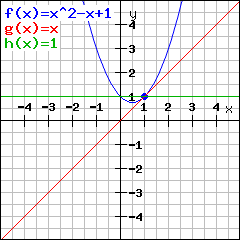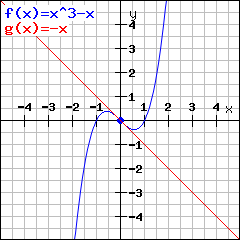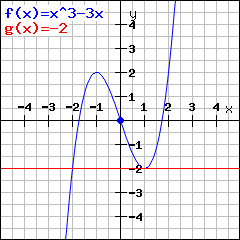# Tangent linesEnter your function here. Hint: Enteras 3*x^2 ,as 3/5 andas (x+1)/(x-2x^4) Enter the point (or the x-position) where you want to get the tangent line.

## What is a tangent line?

A tangent line is a line that just touches something without intersecting it. For example, if you put a ball on the ground, it does just touch the ground, but does not intersect it. So the ground would be a tangent to the ball.
Here are two examples:The green line is no tangent cause the line intersects the graph without just touching it. The read line is a tangent cause it just touches the graph in one point without intersecting it.Mind the special case: A tangent line in an ininflection point does cross the graph of the function. Anyway, the red line is obviously the tangent in the point (0|0), having the same slope as the graph.Also think about this: A line being tangent in one point may very well intersect the graph in some other point.

## Why should I search tangents of function graphs?

If you find a tangent to a graph in a point, you can say that the graph has the same slope as the tangent. So tangents are used to be able to talk about the slope of a graph.

## How to calculate a tangent?

If you want to find the tangent on the point x, you do three things:
• Insert x into the function, so you got the point where the tangent touches
• Insert x into the derivation, so you got the slope m of the tangent.
• Insert m and the point into, then you got b

## Can I see some examples?

Of course. Just enter your function and a point into our free calculator. The tangent will then be found step-by-step.